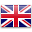# Mathematics

Teaching and learning of Mathematics at OLOR is based on the NSW Syllabus for Mathematics

Students appreciate Mathematics as an essential and relevant part of life and develop their ability to work mathematically.

We encourage positive attitudes towards learning by developing students’ understanding for applying Mathematics effectively to become proficient problem solvers and to value the place of Mathematics in society.

Through our Mathematics program students will:

• develop a sound understanding of mathematical concepts, processes and strategies and the capacity to use these in solving problems
• develop the ability to apply their mathematics to analyse situations and solve real life problems
• develop appropriate language for the effective communication of mathematical ideas and experiences
• develop an appreciation of the applications to mathematics of technology, including calculators and computers

Our Lady of the Rosary Catholic Primary School has mandatory assessment requirements and student tracking in Mathematical learning, from Kindergarten to Year Six. Consistent assessment data analysis from teachers provides valuable information to continually develop student learning from their point of need in Mathematics.English